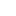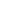# Kruskal’s Algorithm – Minimum Spanning Tree (MST) – Complete Java Implementation

What is Kruskal Algorithm?

• Kruskal’s algorithm for finding the Minimum Spanning Tree(MST), which finds an edge of the least possible weight that connects any two trees in the forest
• It is a greedy algorithm.
• It finds a subset of the edges that forms a tree that includes every vertex, where the total weight of all the edges in the tree is minimized.
• If the graph is not connected, then it finds a minimum spanning forest (a minimum spanning tree for each connected component).
• Number of edges in MST: V-1 (V – no of vertices in Graph).

Example:How this algorithm works?

We strongly recommend reading Find Cycle in Undirected Graph using Disjoint Set (Union-Find) before continue.

1. Sort the edges in ascending order of weights.
2. Pick the edge with the least weight. Check if including this edge in spanning tree will form a cycle is Yes then ignore it if No then add it to spanning tree.
3. Repeat the step 2 till spanning tree has V-1 (V – no of vertices in Graph).
4. Spanning tree with least weight will be formed, called Minimum Spanning Tree

Pseudo Code:

```KRUSKAL(G):
A = ∅
foreach v ∈ G.V:
MAKE-SET(v)
foreach (u, v) in G.E ordered by weight(u, v), increasing:
if FIND-SET(u) ≠ FIND-SET(v):
A = A ∪ {(u, v)}
UNION(u, v)
return A
```

See the animation below for more understanding.JavaCode:

Output:

```Minimum Spanning Tree:
Edge-0 source: 1 destination: 2 weight: 1
Edge-1 source: 1 destination: 3 weight: 2
Edge-2 source: 3 destination: 4 weight: 2
Edge-3 source: 0 destination: 2 weight: 3
Edge-4 source: 4 destination: 5 weight: 6
```

Reference – Wiki

### 5 thoughts on “Kruskal’s Algorithm – Minimum Spanning Tree (MST) – Complete Java Implementation”

1.how i can do this code with Scanner ((user input)) ??

2.In line no. 32 wht does it mean Comparator.comparingInt(o -> o.weight) ?

•Hello Anju,

This line representing “Overriding the Comparator of Priority Queue”, since priority queue is of class “Edge” and it has to be sorted based on the weight of the edges. This is Lamba expression(->)representation in java. You can use the traditional way of doing it as well. Please read the article below for more understanding.

https://algorithms.tutorialhorizon.com/priority-queue-in-data-structure/

••